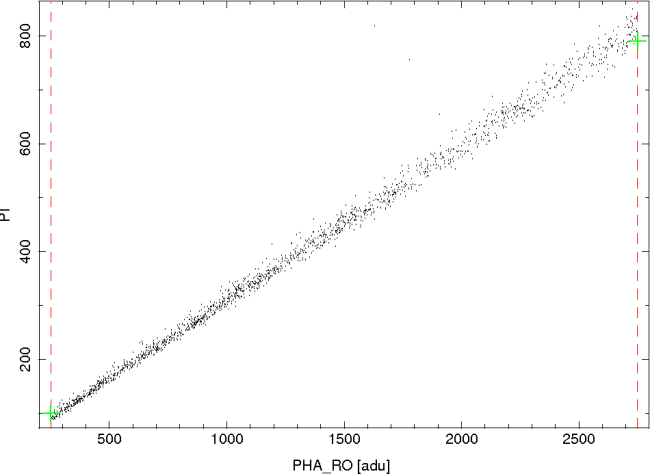## Caveat: Spectral analyses of ACIS data with a limited pulse-height range

### Introduction

When performing analyses of ACIS spectral data, it is important to restrict fits to the spectral band over which the data were collected (or to a narrower band). In general, the valid pulse-height ranges are 39-3769 adu for front-illuminated CCDs (ACIS-I, -S0, -S2, -S4, and -S5) and 21-3769 adu for back-illuminated CCDs (ACIS-S1 and -S3). An estimate of the corresponding energy ranges can be obtained by assuming that 1 adu is equal to 4 eV. Therefore, these nominal ranges are broad enough to cover the entire usable energy band. For observations that use these ranges, it is not necessary to restrict the range of the spectral fits. However, some observations have been performed using narrower ranges of pulse heights.

### Determining the pulse-height range

The lower and upper, user-specified, pulse-height limits for an observation are recorded as PHAMIN and PHAMAX, respectively, in the observation-specific mask file (i.e. acisf*msk1.fits). For example:

1. The nominal pulse-height range of 21-3769 adu was used for CCD_ID 7 (i.e. mask7) of OBS_ID 6899:

```unix% dmlist "acisf06899_000N001_msk1.fits[mask7]" data

ROW  SHAPE       COMPONENT  CHIP(CHIPX,CHIPY)  SAMP_CYC  PHAMIN  PHAMAX

1   rectangle       1      [(2,2) (1023,1023)]      1        21     3769
2   rectangle       2      [(1,1) (1024,1024)]      0        21     3769
3   !rectangle      2      [(2,2) (1023,1023)]      1        21     3769
```
2. A nonstandard lower pulse-height limit of 251 adu was used for CCD_ID 3 (i.e. mask3) of OBS_ID 2296.

```unix% dmlist "acisf02296_000N002_msk1.fits[mask3]" data

ROW  SHAPE       COMPONENT  CHIP(CHIPX,CHIPY)  SAMP_CYC  PHAMIN  PHAMAX

1   rectangle       1      [(2,2) (1023,1023)]      1       251     2749
2   rectangle       2      [(1,1) (1024,1024)]      0       251     2749
3   !rectangle      2      [(2,2) (1023,1023)]      1       251     2749
```
3. A nonstandard upper pulse-height limit of 1274 adu was used for CCD_ID 3 (i.e. mask3) of OBS_ID 7334.

```unix% dmlist "acisf07334_000N001_msk1.fits[mask3]" data

ROW  SHAPE       COMPONENT  CHIP(CHIPX,CHIPY)  SAMP_CYC  PHAMIN  PHAMAX

1   rectangle       1      [(2,3) (1023,1022)]      1        39     1274
2   rectangle       2      [(1,1) (1024,1024)]      0        39     1274
3   !rectangle      2      [(2,3) (1023,1022)]      1        39     1274
```

While it is not necessary to restrict the pulse-height range for spectral analyses of the data in example 1, the pulse-height ranges should be restricted in examples 2 and 3.

Here, the term pulse height is used to refer to PHA, PHA_RO, or PI. By default, the values of PHA and PI are modified to include the CTI and time-dependent gain adjustments. The values of PHA_RO have not been adjusted for these effects. While spectra and RMFs can be binned on PHA or PI, the pulse-height limits are applied to PHA_RO. Therefore, it is necessary to determine the values of PHA or PI that correspond to the limits on PHA_RO. As an example, consider a source at (X, Y) = (4096, 4096) for OBS_ID 2296 (i.e. example 2). The limits on PI for such a source can be estimated by plotting the values of PI v. PHA_RO:

```unix% dmcopy "acisf02296N002_evt2.fits[sky=circle(4096,4096,300)][cols pha_ro,pi]" tmp.fits clobber=yes
unix% ds9 -prism tmp.fits
```Figure 1. A comparison of PI and PHA_RO for the events in a circular region about the aimpoint for OBS_ID 2296. The observation was performed using limits on PHA_RO of 251 and 2749 adu (dashed red lines). Analyses of the PI spectrum of a source in this region should be limited to the range from about 100 to 790 (green plus symbols).

The two vertical dashed red lines in Figure 1 mark the pulse-height limits on PHA_RO at 251 and 2749 adu. As shown, there is a range of values of PI at each limit. The largest value of PI associated with the lower limit is about 100 and the smallest value of PI associated with the upper limit is about 790 (see the green plus symbols in Figure 1). Therefore, analyses of the PI spectrum of this source should be restricted to the range of about 100-790. If PHA spectra are used instead of PI spectra, then "pi" should be replaced with "pha" in the prism command. Likewise, if the location of the source is not at (X, Y) = (4096, 4096), then the coordinates should be replaced by the coordinates of the source. Note that the radius of the circle centered on these coordinates was arbitrarily chosen to be 300 pixels. This radius is large enough to obtain a sufficient number of events to see the distribution of PI v. PHA_RO. Larger values can be used if there are an insufficient number of events in the distribution. However, if the radius becomes too large, then the distribution may be artificially broadened by including regions that have significantly different CTI properties.

### Selecting a limited pulse-height range

Once the PHA or PI limits have been determined, the following commands can be used to restrict the pulse-height range for spectral fits. If the PI limits are 100-790 (example 2), then

• In Sherpa, type

```set_analysis("chan")
ignore("0:99, 791:")
```
• In XSPEC, type

```ignore 0-99 791-**
```
• In ISIS, type

```xnotice_en( 1, 1.4453999996, 11.5340003967 );
```

where the limits are specified in energy (keV) instead of PI. These energies can be obtained from the corresponding RMF by typing

```unix% dmlist "2296.rmf[ebounds]" data

ROW   CHANNEL   E_MIN              E_MAX

.
.
.

99      99     1.4307999611       1.4453999996
100     100     1.4453999996 ***   1.4600000381
101     101     1.4600000381       1.4745999575

.
.
.

789     789     11.5047998428      11.5193996429
790     790     11.5193996429      11.5340003967 ***
791     791     11.5340003967      11.5486001968

.
.
.
```

Note that the same commands should also be used for PHA spectra, although the numerical values of the limits will differ from the values used here.

Any questions or concerns about pulse-height limits should be directed to the CXC Helpdesk.